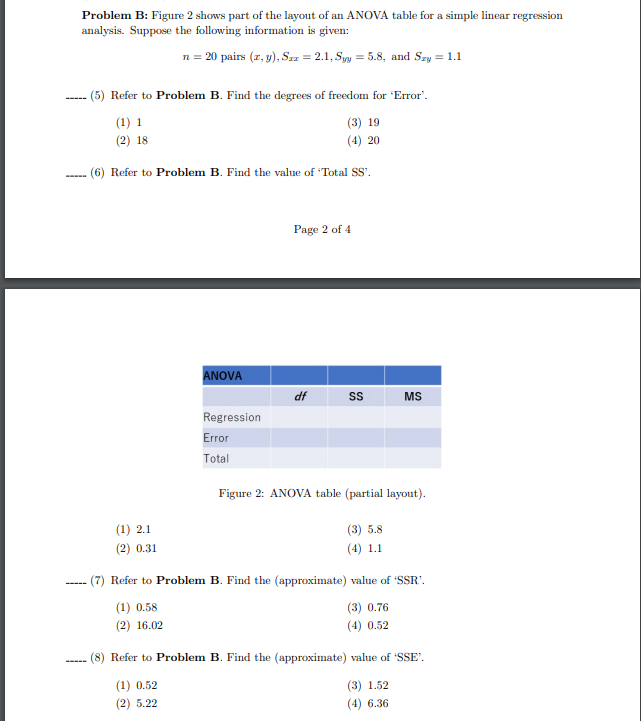Home / Expert Answers / Statistics and Probability / problem-b-problem-b-figure-2-shows-part-of-the-layout-of-an-anova-table-for-a-pa516

# (Solved): problem B Problem B: Figure 2 shows part of the layout of an ANOVA table for a ...

problem B???????

Problem B: Figure 2 shows part of the layout of an ANOVA table for a simple linear regression analysis. Suppose the following information is given: pairs , and (5) Refer to Problem B. Find the degrees of freedom for 'Error'. (1) 1 (3) 19 (2) 18 (4) 20 (6) Refer to Problem B. Find the value of 'Total SS'. Page 2 of 4 Figure 2: ANOVA table (partial layout). (1) (3) (2) (4) (7) Refer to Problem B. Find the (approximate) value of 'SSR'. (1) (3) (2) (4) (8) Refer to Problem B. Find the (approximate) value of 'SSE'. (1) (3) (2) (4)

We have an Answer from Expert